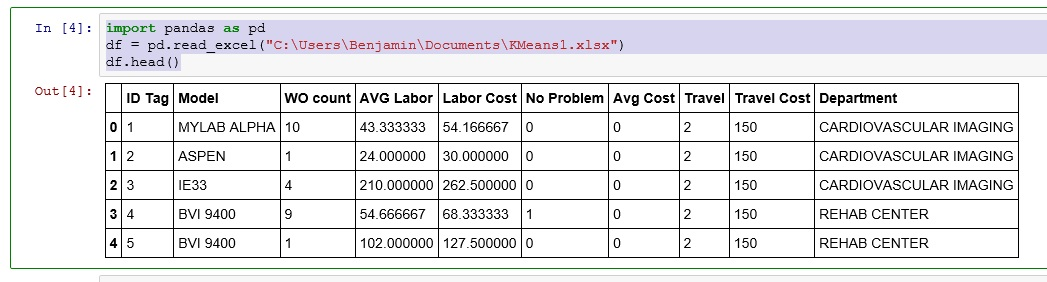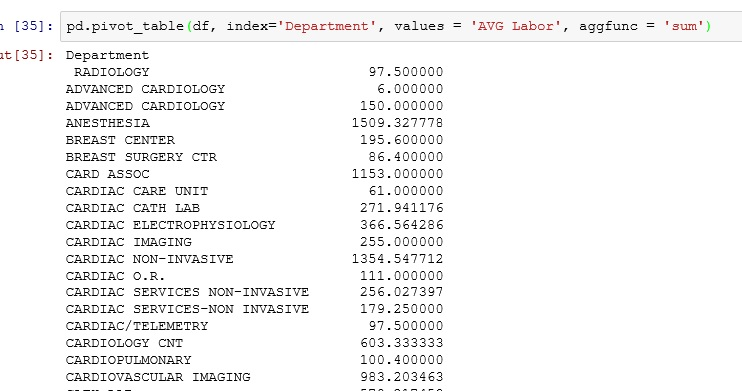# Python: Pivot Tables with Pandas

Pandas provides Python with lots of advanced data management tools. Being able to create pivots tables is one of the cooler tools.

You can download the data set I will be working with here: KMeans1

First, let’s upload our data into Python

```import pandas as pdLet’s create our first pivot table.

The syntax is = pd.pivot_table(dataframe, index = Columns you want to group by, values = Columns you want to aggregate, aggfunc = type of aggregation)

`pd.pivot_table(df, index='Department', values = 'AVG Labor', aggfunc = 'sum')`We can group by more than one column

```pd.pivot_table(df, index=['Department','Model'], values = 'AVG Labor',
aggfunc= 'mean')```You can also have multiple value columns

``` pd.pivot_table(df, index='Department', values = ['AVG Labor','Labor Cost'],
aggfunc= 'sum')```Now, one catch is, what if you want to have different aggregate functions. What if I want to get the mean of AVG Labor, but I want the sum of Labor Cost columns.

In this case we are going to use the groupby().aggregate()

```import numpy as np
df.groupby('Department').aggregate({'AVG Labor':np.mean, 'Labor Cost': np.sum})```## One thought on “Python: Pivot Tables with Pandas”

1.Kabir Ajibade

I’m definitely trying this out, thanks for the tip